Question
singal and system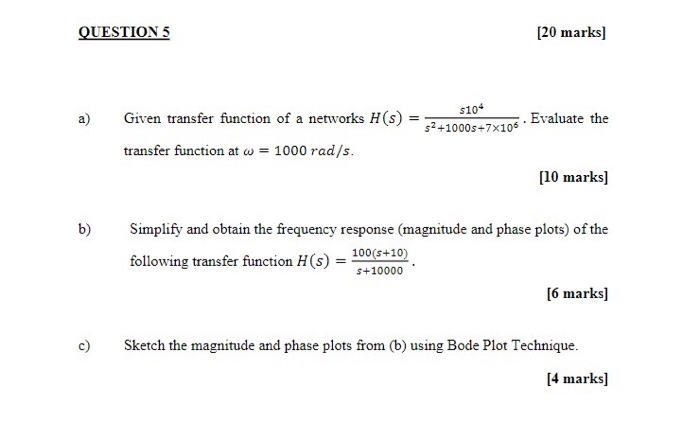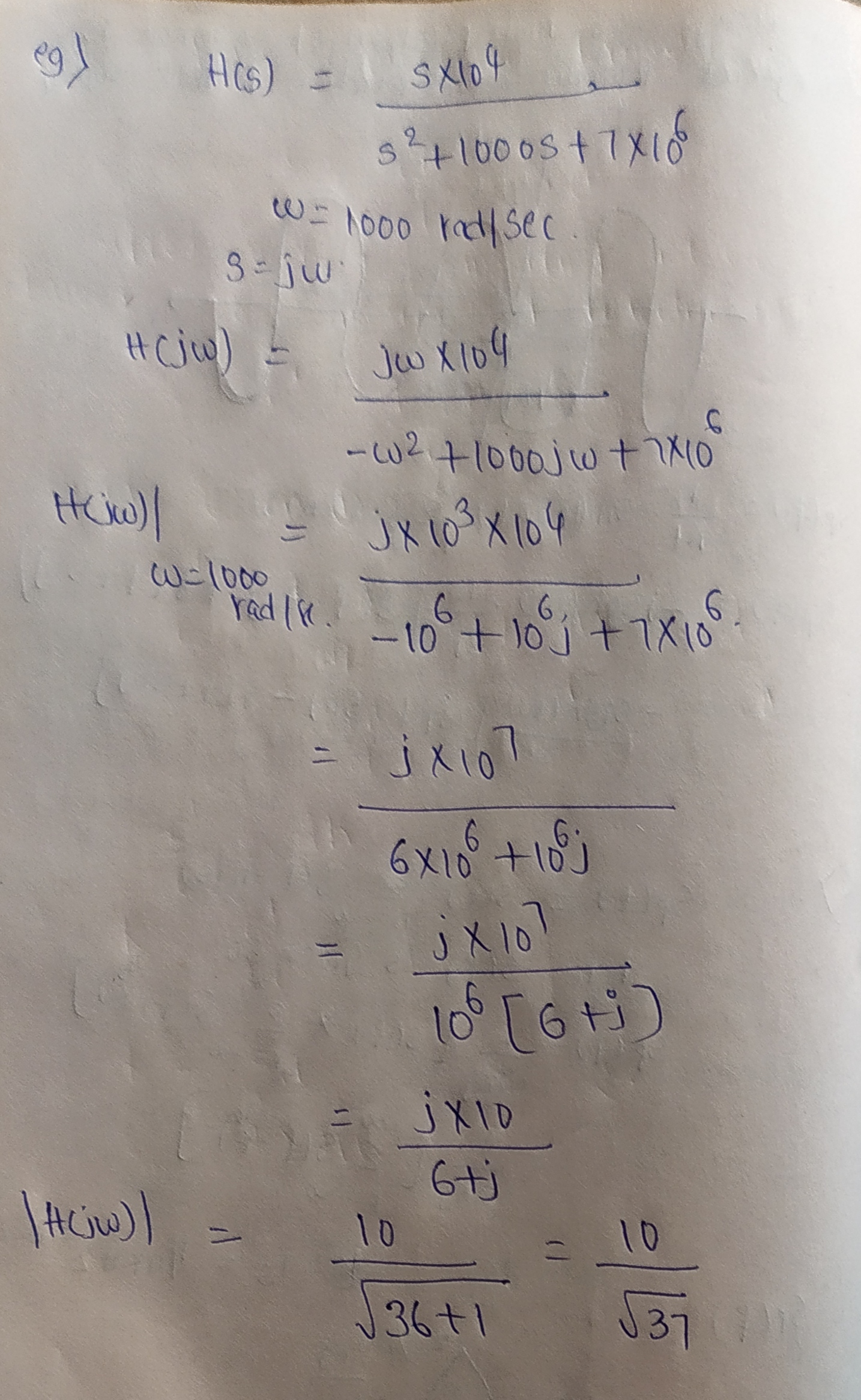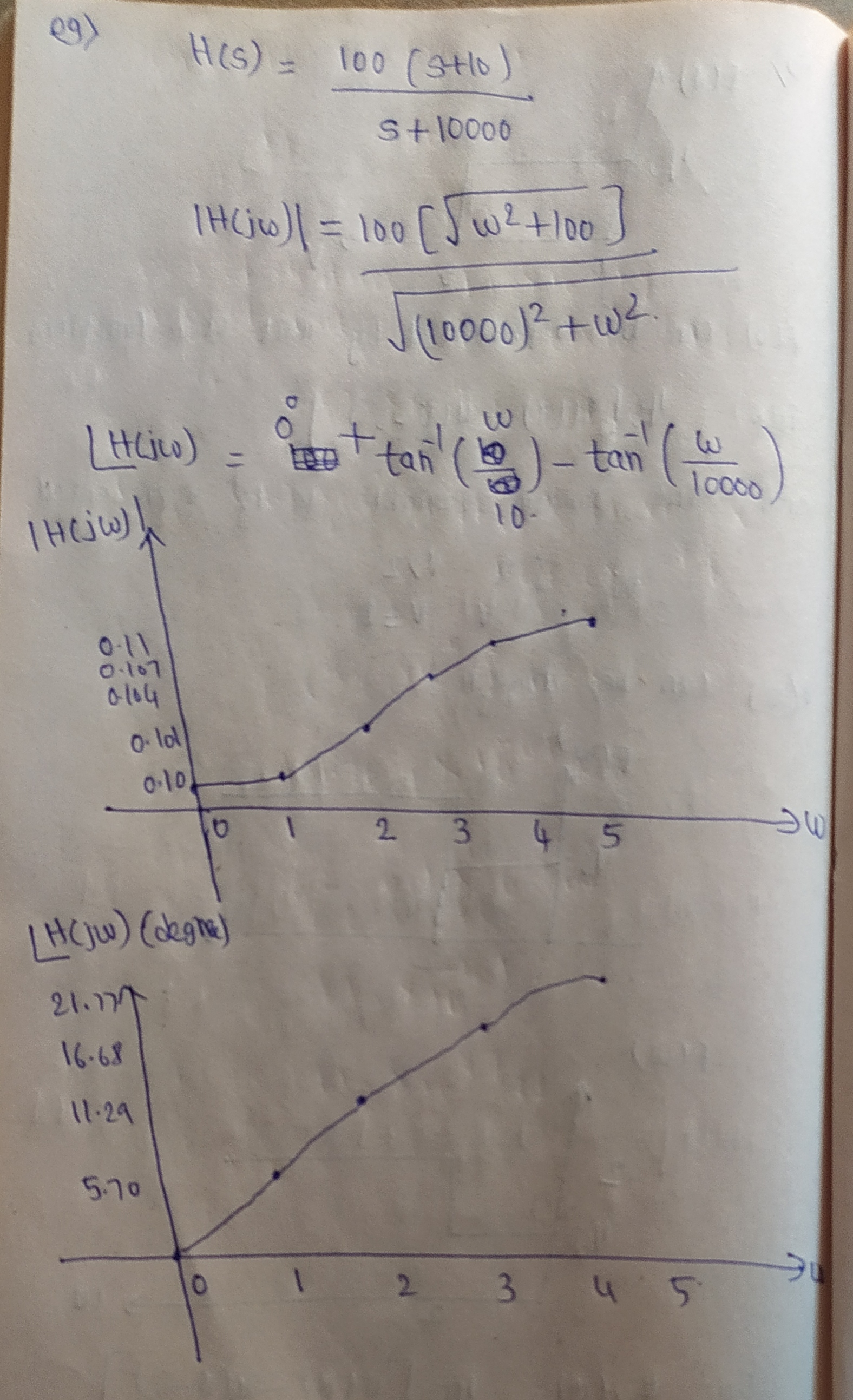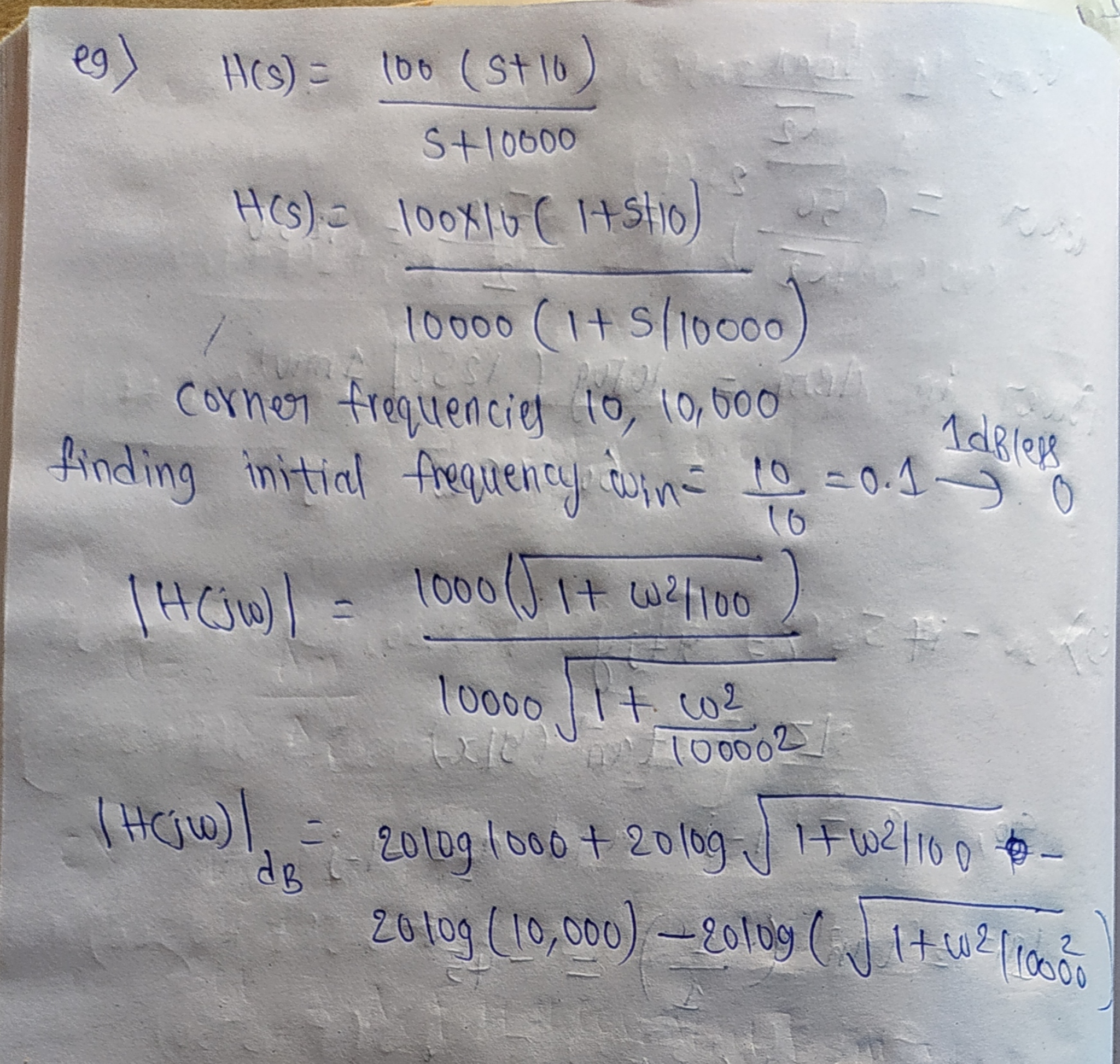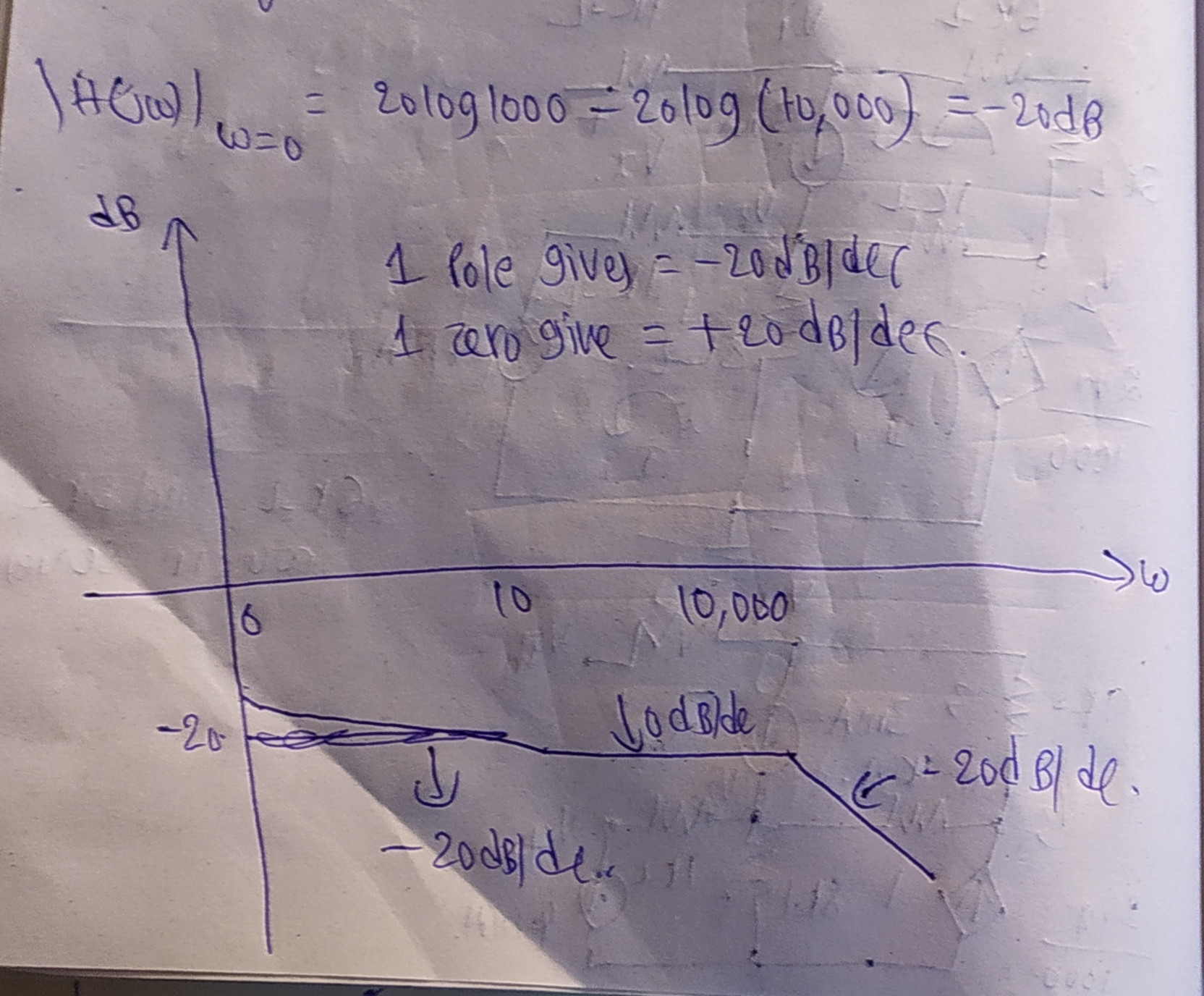#### Earn Coins

Coins can be redeemed for fabulous gifts.

Similar Homework Help Questions
• ### Please answer all parts Problem #5 - (20%) A circuit has the transfer function: H(S) =...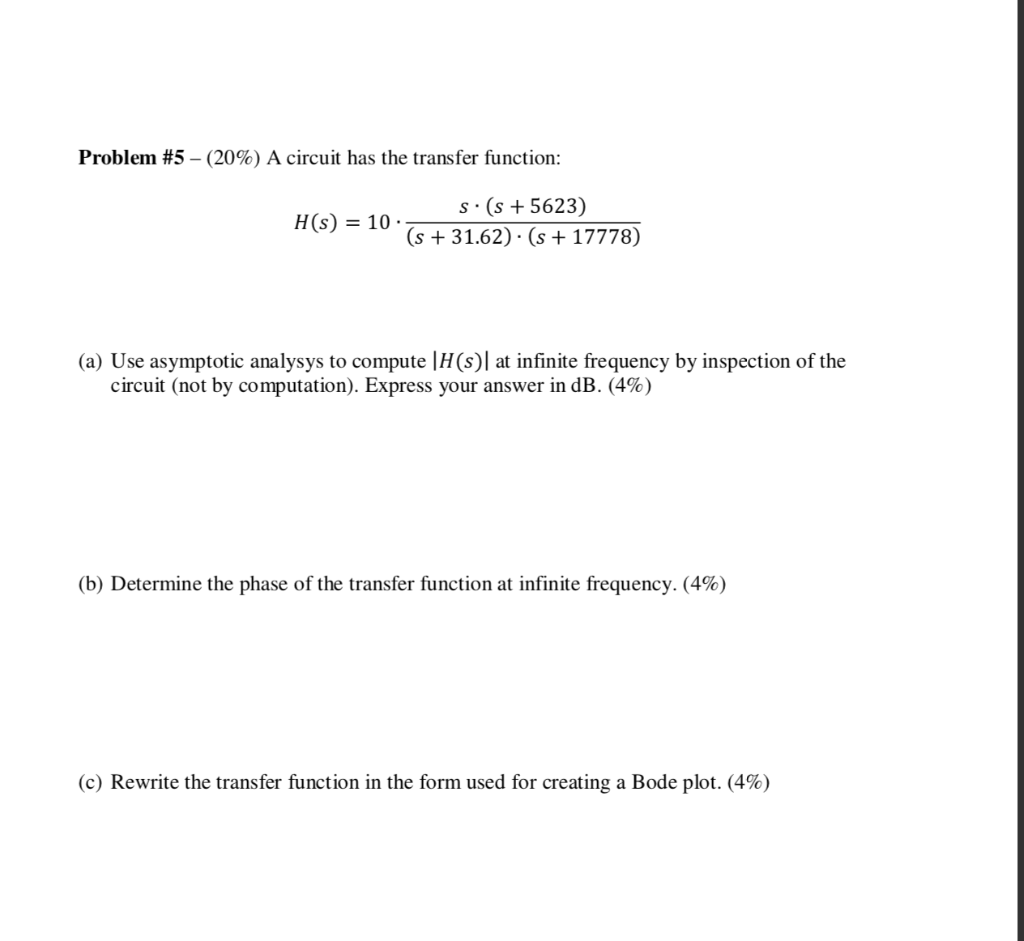Please answer all parts Problem #5 - (20%) A circuit has the transfer function: H(S) = S. (s + 5623 (s + 31.62) · (s + 17778) (a) Use asymptotic analysys to compute (HS) at infinite frequency by inspection of the circuit (not by computation). Express your answer in dB. (4%) (b) Determine the phase of the transfer function at infinite frequency. (4%) (c) Rewrite the transfer function in the form used for creating a Bode plot. (4%) Problem #5...

• ### 1. (20 points). A transfer function has the following zeros and poles: zero at s=-105 and s= poles at s-100 and s--1000. The magnitude of the transfer function at ω= 105 rad/s is equal 100. Find the...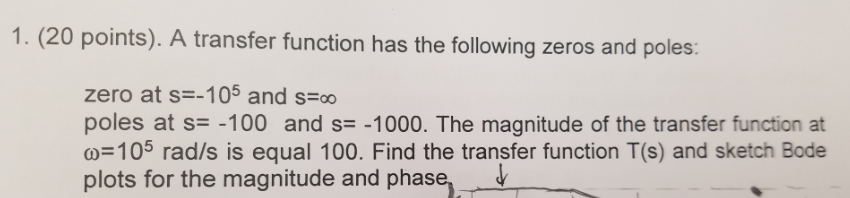1. (20 points). A transfer function has the following zeros and poles: zero at s=-105 and s= poles at s-100 and s--1000. The magnitude of the transfer function at ω= 105 rad/s is equal 100. Find the transfer function T(s) and sketch Bode plots for the magnitude and phase, ˇ 1. (20 points). A transfer function has the following zeros and poles: zero at s=-105 and s= poles at s-100 and s--1000. The magnitude of the transfer function at ω=...

• ### 105- Problem #4 - Given the transfer function T(s)- ***(1+%o1+%0011+%00) A) Find the Poles and Zeros....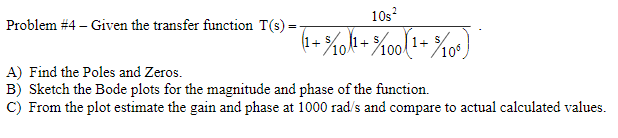105- Problem #4 - Given the transfer function T(s)- ***(1+%o1+%0011+%00) A) Find the Poles and Zeros. B) Sketch the Bode plots for the magnitude and phase of the function. C) From the plot estimate the gain and phase at 1000 rad/s and compare to actual calculated values.

• ### 1. A unity feedback system has open-loop transfer function given by an 100 G(s)s2)(s +4) a....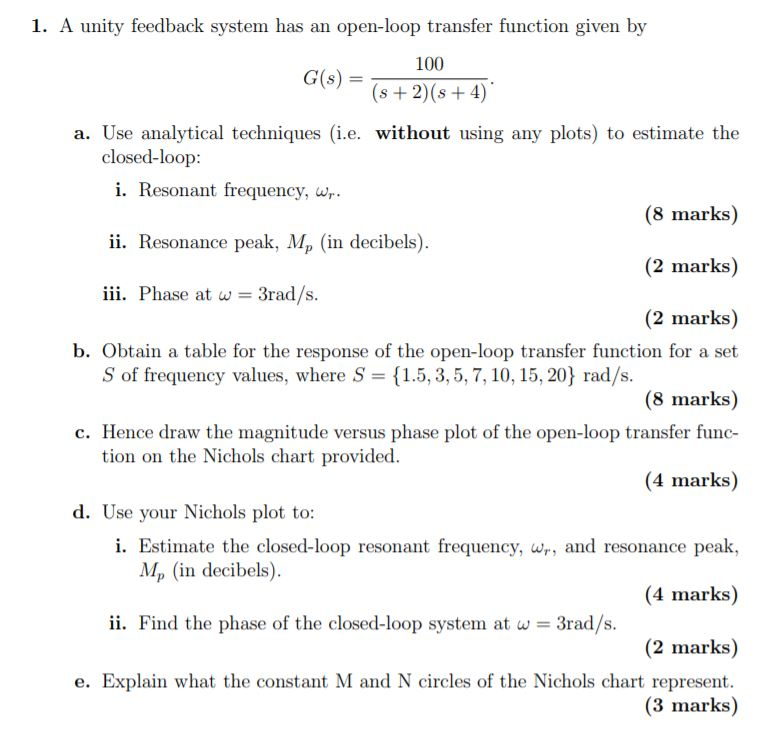1. A unity feedback system has open-loop transfer function given by an 100 G(s)s2)(s +4) a. Use analytical techniques (i.e. without using any plots) to estimate the closed-loop: i. Resonant frequency, w (8 marks) ii. Resonance peak, Mp (in decibels) (2 marks) i. Phase at w = 3rad/s (2 marks) b. Obtain a table for the response of the open-loop transfer function for a set S of frequency values, where S {1.5,3,5,7, 10, 15, 20} rad/s (8 marks) Hence draw...

• ### Let a transfer function H be 1000s + 10) 100+1000 Use H to respond to the following questions and...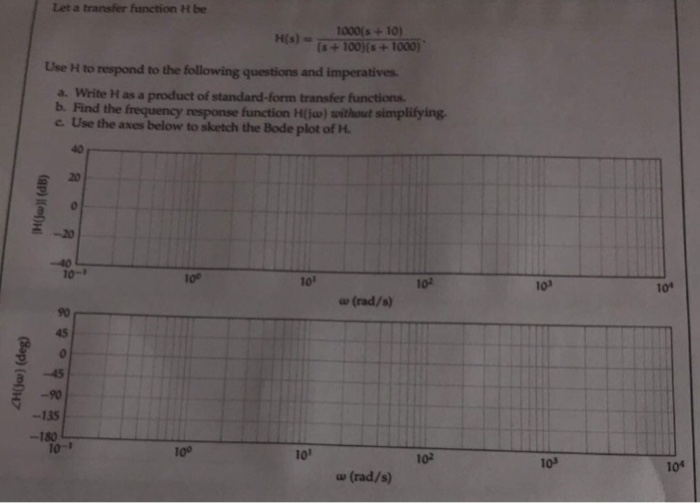System dynamics course. Let a transfer function H be 1000s + 10) 100+1000 Use H to respond to the following questions and imperatives a. Write H as a product of standard-form transfer functions Find the frequency response function H(jaw) without simplifying c. Use the axes below to sketch the Bode plot of H. 20 -20 10-1 10° 101 102 103 10 w (rad/s) 90 45 45 -90 -135 -180 10-T 100 101 102 103 101 w (rad/s) Let a transfer...

• ### For the given transfer function: Ho-2where s 5 (s s (s +10) where s =j w...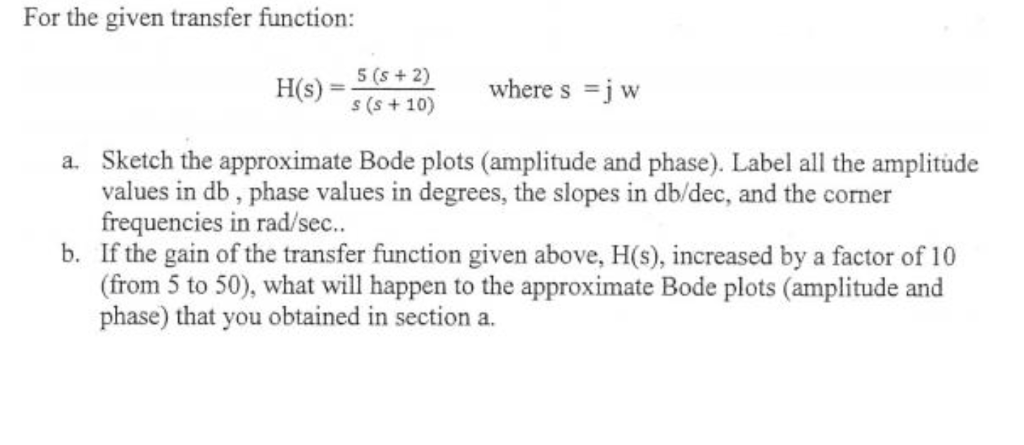For the given transfer function: Ho-2where s 5 (s s (s +10) where s =j w Sketch the approximate Bode plots (amplitude and phase). Label all the amplitude values in db, phase values in degrees, the slopes in db/dec, and the corner frequencies in rad/sec.. a. b. If the gain of the transfer function given above, H(s), increased by a factor of 10 (from 5 to 50), what will happen to the approximate Bode plots (amplitude and phase) that you...

• ### 1. A unity feedback system has open-loop transfer function given by an 100 G(s)s2)(s +4) a....1. A unity feedback system has open-loop transfer function given by an 100 G(s)s2)(s +4) a. Use analytical techniques (i.e. without using any plots) to estimate the closed-loop: i. Resonant frequency, w (8 marks) ii. Resonance peak, Mp (in decibels) (2 marks) i. Phase at w = 3rad/s (2 marks) b. Obtain a table for the response of the open-loop transfer function for a set S of frequency values, where S {1.5,3,5,7, 10, 15, 20} rad/s (8 marks) Hence draw...

• ### I need help with this  Bode Plots assignment 2. (5 points) Follow the steps (a-c) described below for the following tra...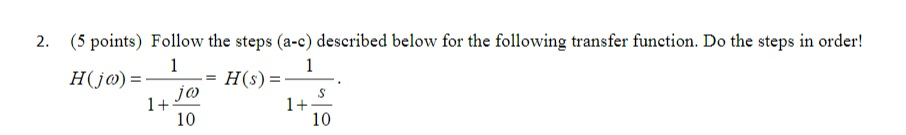I need help with this  Bode Plots assignment 2. (5 points) Follow the steps (a-c) described below for the following transfer function. Do the steps in order! 1+ 10 1+ 10 a. On a 'blank Bode plot' grid, plot the Bode plot straight line hand sketch approximation for magnitude for the frequency range from 1000 to ω.*1000. Be sure, though, that the corner frequency aligns with an appropriate vertical line on the grid. b. On graph paper, directly below the...

• ### I need help with this  Bode Plots assignment (5 points) Follow the steps (a-c) described below for the following transf...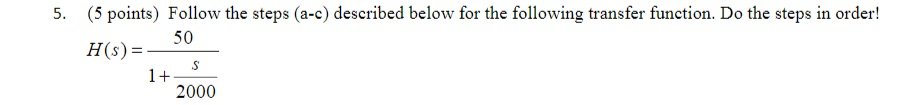I need help with this  Bode Plots assignment (5 points) Follow the steps (a-c) described below for the following transfer function. Do the steps in order! 5. H50 H(s) 2000 a. On a 'blank Bode plot' grid, plot the Bode plot straight line hand sketch approximation for magnitude for the frequency range from 1000 to ω.*1000. Be sure, though, that the corner frequency aligns with an appropriate vertical line on the grid. b. On graph paper, directly below the magnitude...

• ### For the following closed-loop transfer functions, sketch the bode plots (magnitude and phase), id...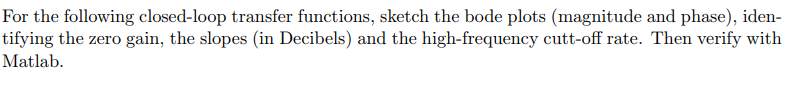For the following closed-loop transfer functions, sketch the bode plots (magnitude and phase), iden- tifying the zero gain, the slopes (in Decibels) and the high-frequency cutt-off rate. Then verify with Matlab (6) wn = 1, 〈 0.0.1, and 0.707. (8) Assuming the system of Problem 6 above, and an input of r(t) = 30sin(1000 t), use your bode plot to obtain the steady-state response For the following closed-loop transfer functions, sketch the bode plots (magnitude and phase), iden- tifying the...